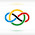### 2015 Azerbaijan National Olympiad #2

Let $a,b$ and $c$ be the length of sides of a triangle. Then prove that $S<\frac{a^2+b^2+c^2}{6}$ where $S$ is the area of triangle.

Let $a\le b\le c$. $$a^2+b^2\ge2ab=4\cdot\frac{1}{2}ab\ge4\cdot\frac{1}{2}ab\cdot\sin C=4S$$$$\frac{a^2+b^2}{4}\ge S$$$$\frac{a^2+b^2+c^2}{6}\ge\frac{a^2+b^2}{4}\ge S$$However there cannot be equality, as if $\sin C=1$, then we cannot have $a=b=c$.

We are done. $\square$

1.mahaler12/30/2021

I always love inequalities :)

Nice solution! :D

2.@mahaler

Thanks :)